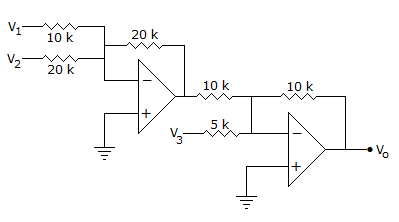# Electronics and Communication Engineering - Measurements and Instrumentation

### Exercise :: Measurements and Instrumentation - Section 6

1.

The Y plates of a CRO are excited by a voltage 2 sin 100 t and the X plates are not connected. The display would be

 A. a vertical line B. a horizontal line C. sine wave D. slant line

Explanation:

No answer description available for this question. Let us discuss.

2.

Heating effect of current is used in

 A. ammeters B. voltmeters C. both ammeters and voltmeters D. wattmeters

Explanation:

No answer description available for this question. Let us discuss.

3.

The coils of Drysdale polar potentiometer are wound non-inductively.

 A. True B. False

Explanation:

No answer description available for this question. Let us discuss.

4.

In figure the output V0 isA. 2V1 + V2 - 2V3 B. 2(V1 + V2) - V3 C. V1 + V2 + V3 D. V1 + 2V2 - V3

Explanation:

No answer description available for this question. Let us discuss.

5.

Magnetic effect of current is used in

 A. ammeters B. voltmeters C. wattmeters D. ammeters, voltmeters, wattmeters and energy meters